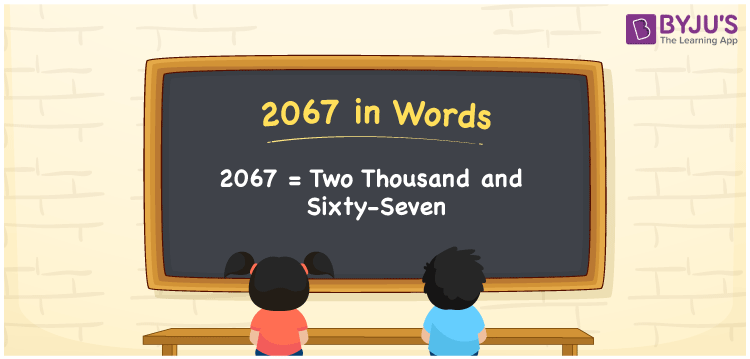# 2067 in Words

The number 2067 in words is represented as Two Thousand and Sixty-Seven. For instance, the cost of a study lamp is Rs. 2067, then you can say, “The cost of a study lamp is Rupees Two Thousand and Sixty-Seven”. 2067 is a cardinal number. It is easy to convert any number into words using a place value chart. Hence, it is essential to use a place value chart to represent numerical names. Here, we will learn how to convert 2067 into words along with its interesting facts in detail.

 2067 in Words Two Thousand and Sixty-Seven Two Thousand and Sixty-Seven in numerical form 2067

## 2067 in English Words

Generally, we write numbers in words with the help of the English alphabet. Therefore, we can read the number 2067 in English as Two Thousand and Sixty-Seven.## How to Write 2067 in Words?

First, identify the place values of each digit before converting it into the word form. The place value chart for 2067 and its expanded form is given below.

 Thousands Hundreds Tens Ones 2 0 6 7

Hence, we can write the expanded form as:

2 x Thousand + 0 x Hundred + 6 x Ten + 7 x One

= 2 x 1000 + 0 x 100 + 6 x 10 + 7 x 1

= 2000 + 0 + 60 + 7

= 2000 + 60 + 7

= 2067

= Two Thousand and Sixty-Seven

Therefore, 2067 in words is written as Two Thousand and Sixty-Seven

Interesting way of writing 2067 in words

2 = Two

20 = Twenty

206 = Two Hundred and Six

2067 = Two Thousand and Sixty-Seven

Thus, the word form of the number 2067 is Two Thousand and Sixty-Seven

2067 is a natural number that precedes 2068 and succeeds 2066

• 2067 in words – Two Thousand and Sixty-Seven
• Is 2067 an odd number? – Yes
• Is 2067 an even number? – No
• Is 2067 a perfect square number? – No
• Is 2067 a perfect cube number? – No
• Is 2067 a prime number? – No
• Is 2067 a composite number? – Yes

## Frequently Asked Questions on 2067 in Words

Q1

### How do you write 2067 in English words?

2067 in English words is written as Two Thousand and Sixty-Seven.
Q2

### What is the place value of 6 in 2067?

The place value of 6 in 2067 is Tens.
Q3

### Convert Two Thousand and Sixty-Seven in numbers.

Two Thousand and Sixty-Seven in numbers is 2067.